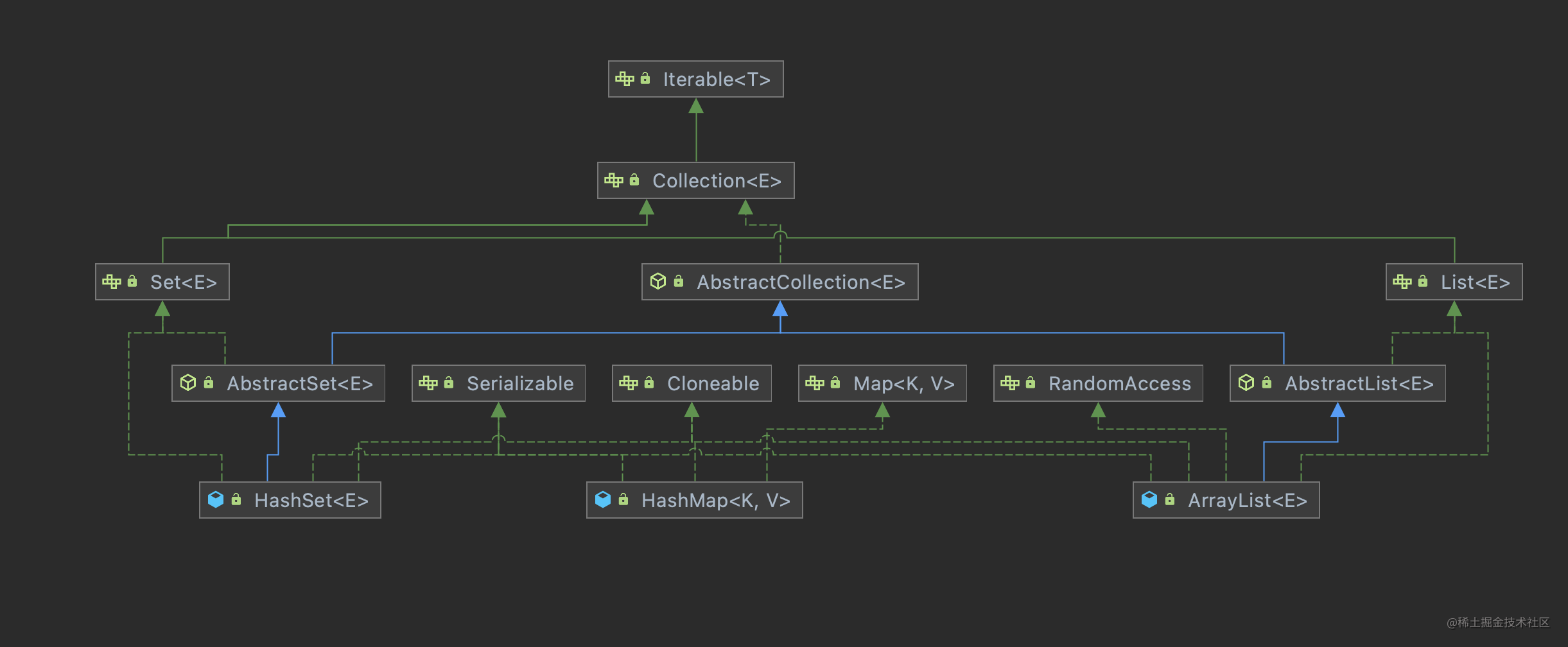# Kotlin - 集合类

``````val funList: List<(Int) -> Boolean> = listOf(
{ it -> it % 2 == 0 }, // 第一个函数为 { it -> it % 2 == 0 }
{ it -> it % 2 == 1 }) // 第二个函数为 { it -> it % 1 == 1}

``````fun main() {
val list = listOf(1, 2, 3, 4, 5, 6, 7)

list.filter(funList) // [2, 4, 6]
list.filter(funList) // [1, 3, 5, 7]
}

### 集合类概述

Kotlin 的集合类分为：可变集合类（Mutable）和 不可变集合类 （Immutable）。### 不可变集合类

List 列表分为只读不可变的 List 和可变 MutableList(可写入、删除数据)。 Set 集也分为不可变 Set 和可变 MutableSet(可写入、删除数据)。 Map 也分为只读 Map 和可变 MutableMap(可写入、删除数据)。

### 创建集合类

Kotlin 中分别使用 listOf()、setOf()、mapOf() 函数创建不可变的 List 列表容器、Set 集容器、Map 映射容器；使用 mutableListOf()、mutableSetOf()、mutableMap() 函数来创建可变的 MutableList 列表容器、MutableSet 集容器、MutableMap 映射容器。

``````val list = listOf(1, 2, 3, 4, 5, 6, 7)  // 创建不可变的list
val mutableList = mutableListOf("a", "b", "c")  //创建可变MutableList

val set = setOf(1, 2, 3, 4, 5, 6, 7)    // 创建不可变 Set
val mutableSet = mutableSetOf("a", "b", "c") //创建可变的MutableSet

val map = mapOf(1 to "a", 2 to "b", 3 to "c") //创建不可变Map
val mutableMap = mutableMapOf(1 to "X", 2 to "Y", 3 to "Z")

### 遍历集合中的元素

List、Set 类继承了 Iterable 接口，里面扩展了 forEach 函数来迭代遍历元素；同样，Map 接口中也扩展了 forEach 函数来迭代遍历元素。

``````list.forEach {
println(it)
}

set.forEach {
println(it)
}

map.forEach {
println("K = \${it.key}, V = \${it.value}")
}

``````@kotlin.internal.HidesMembers
public inline fun <T> Iterable<T>.forEach(action: (T) -> Unit): Unit {
for (element in this) action(element)
}

@kotlin.internal.HidesMembers
public inline fun <K, V> Map<out K, V>.forEach(action: (Map.Entry<K, V>) -> Unit): Unit {
for (element in this) action(element)
}

``````list.forEachIndexed { index, value -> // 带下标 index 来遍历 List
println("list index = \${index}, value = \$value")
}

set.forEachIndexed { index, value -> // 带下标 index 来遍历 Set
println("set index = \${index}, value = \${value}")
}

``````public inline fun <T> Iterable<T>.forEachIndexed(action: (index: Int, T) -> Unit): Unit {
var index = 0
for (item in this) action(checkIndexOverflow(index++), item)
}

### 映射函数

``````val list = listOf(1, 2, 3, 4, 5, 6, 7)
val set = listOf(1, 2, 3, 4, 5, 6, 7)
val map = mapOf(1 to "a", 2 to "b", 3 to "c")

list.map { it * it } // map 函数对每个元素进行乘方操作，返回 [1, 4, 5, 16, 25, 36, 49]
set.map { it + 1 } // map 函数对每个元素进行加 1 操作，返回 [2, 3, 4, 5, 6, 7, 8]
map.map { it.value + "\$" } // map 函数对每个元素加上字符 \$，返回 [a\$, b\$, c\$]

map 函数的签名如下：

``````public inline fun <T, R> Iterable<T>.map(transform: (T) -> R): List<R>
public inline fun <K, V, R> Map<out K, V>.map(transform: (Map.Entry<K, V>) -> R): List<R>

``````val strList = listOf("a", "b", "c")
// 每个元素 it 映射之后返回一个 List，这个 List 中有四个元素
// 分别是： it + 1， it + 2, it + 3, it + 4
strList.map { it -> listOf(it + 1, it + 2, it + 3, it + 4) }

``````[[a1, a2, a3, a4], [b1, b2, b3, b4], [c1, c2, c3, c4]]

Kotlin 中还提供了一个 flatten() 函数，效果是把嵌套的 List 结构 “平铺”，变成一层的结构，代码示例如下：

``````strList.map { it -> listOf(it + 1, it + 2, it + 3, it + 4) }.flatten()

``````[a1, a2, a3, a4, b1, b2, b3, b4, c1, c2, c3, c4]

flatMap 函数是 map 和 flat 两个函数的 “复合逻辑”，代码示例如下：

``````flatMap = strList.flatMap { it -> listOf(it + 1, it + 2, it + 3, it + 4) }

``````[a1, a2, a3, a4, b1, b2, b3, b4, c1, c2, c3, c4]

### 过滤函数

``````studentList.filter{ it.age >= 18}

``````val list = listOf(1, 2, 3, 4, 5, 6, 7)
list.filterIndexed { index, it -> index % 2 == 9 && it > 3 } // 返回 [5, 7]

filterIndexed() 函数签名如下：

``````public inline fun <T> Iterable<T>.filterIndexed(predicate: (index: Int, T) -> Boolean): List<T>

### 排序函数

Kotlin 集合类中提供了倒序排列集合元素的函数 reversed()，代码示例如下：

``````val list = listOf(1, 2, 3, 4, 5, 6, 7)
list.reversed() //倒序函数，返回 [7, 6, 5, 4, 3, 2, 1]

``````public fun <T> Iterable<T>.reversed(): List<T> {
val list = toMutableList()
list.reverse()
return list
}

``````list.sorted()

Kotlin 中的这个 sorted() 函数也是直接调用 Java 的 API 来实现的，相关代码如下：

``````public fun <T : Comparable<T>> Iterable<T>.sorted(): List<T> {
if (this is Collection) {
if (size <= 1) return this.toList()
@Suppress("UNCHECKED_CAST")
return (toTypedArray<Comparable<T>>() as Array<T>).apply { sort() }.asList()
}